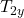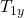## A traffic light is weighing 200N hangs from a vertical cable tied to two other cables that are fastened to a support. The upper cable makes

Question

A traffic light is weighing 200N hangs from a vertical cable tied to two other cables that are fastened to a support. The upper cable makes angles of 41° and 63° with the horizontal. Calculate the tension in each of the three cables.

in progress 0
2 weeks 2021-08-29T00:17:42+00:00 1 Answers 0 views 0

T₁ = 93.6 N
, T₂ = 155.6 N
, T₃ = 200 N

Explanation:

This is a balance exercise where we must apply the expressions for translational balance in the two axes

∑  F = 0

Suppose that cable t1 goes to the left and the angles are 41º with respect to the horizontal and cable t2 goes to the right with angles of 63º

decompose the tension of the two upper cables

cos 41 = T₁ₓ / T1

sin 41 = T₁y / T1

T₁ₓ = T₁  cos 41

T₁y= T₁  sin 41

for cable gold

cos 63 = T₂ / T₂

sin 63 =/ T₂

We apply the two-point equilibrium equation: The junction point of the three cables and the point where the traffic light joins the vertical cable.

Let’s start by analyzing the point where the traffic light meets the vertical cable

T₃ – W = 0

T₃ = W

T₃ = 200 N

now let’s write the equations for the single point of the three wires

X axis

– T₁ₓ + T₂ₓ = 0

T₁ₓ = T₂ₓ

T1 cos 41 = T2 cos 63

T1 = T2 cos 63 / cos 41                (1)

y Axis+ T_{2y} – T3 = 0

T₁ sin 41 + T₂ sin 63 = T₃          (2)

to solve the system we substitute equation 1 in 2

T₂ cos 63 / cos 41 sin 41 + T₂ sin 63 = W

T₂ (cos 63 tan 41 + sin 63) = W

T₂ = W / (cos 63 tan 41 + sin 63)

We calculate

T₂ = 200 / (cos 63 tan 41 + sin 63)

T₂ = 200 / 1,2856

T₂ = 155.6 N

we substitute in 1

T₁ = T₂ cos 63 / cos 41

T₁ = 155.6 cos63 / cos 41

T₁ = 93.6 N

therefore the tension in each cable is

T₁ = 93.6 N

T₂ = 155.6 N

T₃ = 200 N